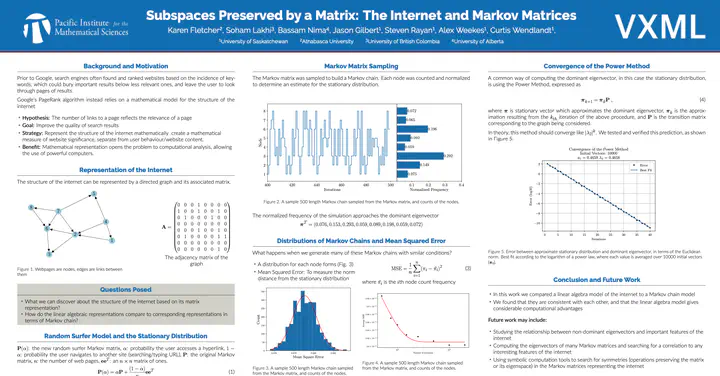# Subspaces Preserved by a MatrixPoster for “Subspaces Preserved by a Matrix”

• Jason Gilbert, University of Saskatchewan

• Karen Fletcher, Athabasca University
• Soham Lakhi, University of British Columbia
• Bassam Nima, University of Alberta

## Problem Statement

One of the most fundamental problems in linear algebra is that of finding the eigenvectors of a given matrix. We can vastly generalize this problem by asking to find all subspaces which are preserved by the matrix. This more general problem is relevant to several applications of linear algebra, such as control theory and statistical inference, and is also theoretically significant. In spite of its apparent simplicity, there are related geometric and algebraic questions which are less well understood. One example which can be probed with computational tools is the question of giving a complete list of algebraic equations encoding the above problem, over a field of arbitrary characteristic – a question with surprising ties to the combinatorics of higher level Fock spaces in conformal field theory and to the topology of certain moduli spaces.

Putting aside the applications, the central problem itself only requires a working knowledge of linear algebra. Students involved in the project will sharpen their understanding of linear algebra and become adept at computations that are less explored in typical undergraduate linear algebra classes.

### Final Report

This VXML project was completed by the participants listed above, as described in the following documents

### Details

• Expected team size: 2
• Student Experience Level: Advanced: students who have taken multiple higher level mathematics courses
##### Prerequisites
• Linear Algebra II or equivalent
##### Skills
• Sage/Jupyter or similar programming tools (preferred but optional)##### Steven Rayan
###### Professor of Mathematics, University of Saskatchewan##### Alex Weekes
###### Assistant Professor of Mathematics, University of Saskatchewan